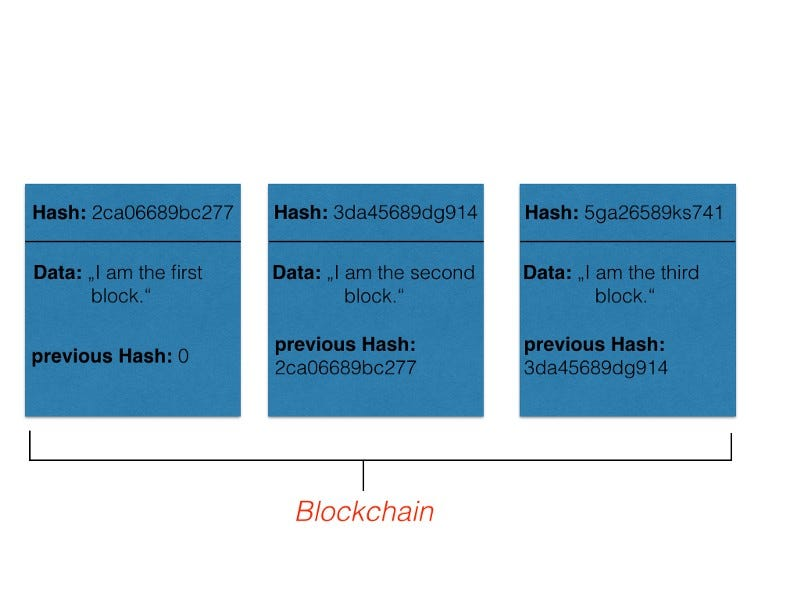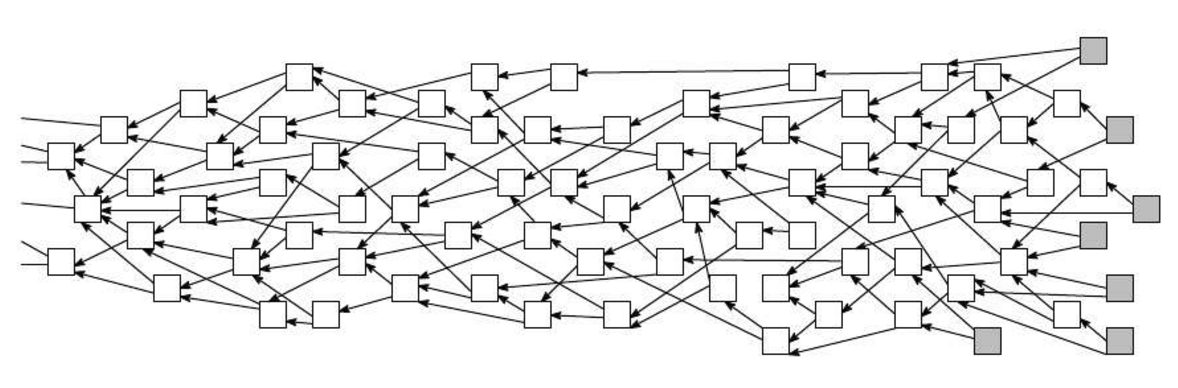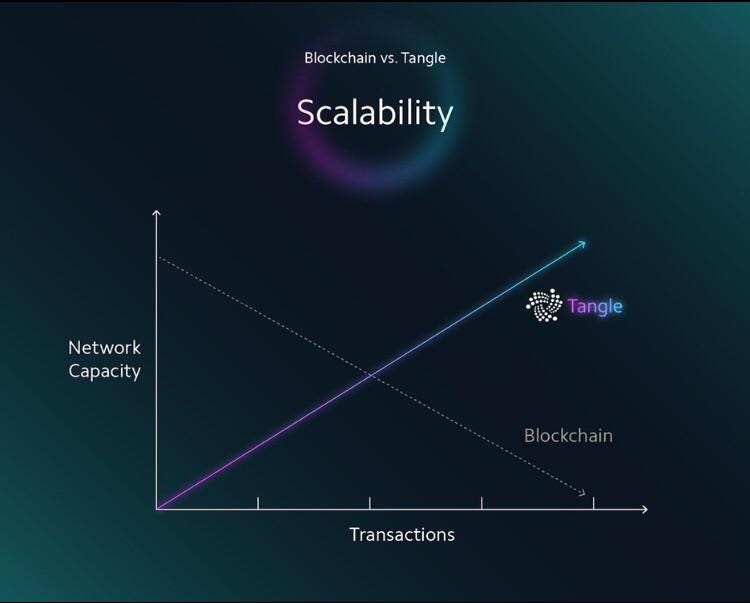# How IOTA solves Blockchains scalability problem

## This article gives a short introduction to blockchain’s scalability problem and how Tangle solves it.https://www.google.de/search?q=tangle+iota&source=lnms&tbm=isch&sa=X&ved=0ahUKEwi_l7-wi-_dAhUMuaQKHU1DDw0Q_AUIDigB&biw=1363&bih=539#imgrc=hEDjkFFOylj9xM:

#### Introduction to the Scalability Problem

Blockchain as a decentralized and cryptographically secured database is one of the new technologies, that will definitely have a huge impact on the future of the digital world. But with an increasing impact comes an increasing number of users — and there a big problem occurs. Most of the currently known blockchain networks are not made for many users — and I mean a lot of users. Let’s just take a look at the most famous blockchain system Bitcoin. The size of a block, which determines the amount of data being able to be stored, is limited to 1MB. As I described in one of my previous articles, How cryptocurrencies actually work, the data stored in cryptocurrency blockchains are transaction data. A block has to be generated and then validated by the network, and this takes time. Thus, there is only a limited amount of transactions, that can be done per a certain amount of time. But since this whole technology gets more and more participants, the time to handle such transactions, increases. This is called the problem of scalability. A solution had to be found, and IOTA did find one.

#### IOTA and the Tangle

The IOTA foundation came up with a pretty nice idea — the so called Tangle system. Therefore they used their knowledge from a topic in maths called Graph theory. No worry, I will explain it. First, take a look at a simplified structure of a blockchain in the figure below:Visualization of a simplified structure of a blockchain.

We have blocks of information, each block contains certain data and is connected to the previous block via a cryptographically calculated hash value. Now take a look at how the tangle looks like:Source: https://www.google.de/search?q=tangle+iota&source=lnms&tbm=isch&sa=X&ved=0ahUKEwjuz_PXgu_dAhUGUlAKHYBLBcsQ_AUIDigB&biw=1363&bih=601#imgrc=cUOYORd9LALTBM:

What you can see in the figure above, is something that is called a Directed Acyclic Graph in mathematics. For the purpose of understanding it is enough to know, that a graph is just a set of vertices and edges. Directed means, that the edge, that connects two vertices, directs from one vertex to the other. In the tangle system, each transaction is represented by a vertex. That means, if someone is in the process of transferring some IOTA funds, he or she generates a transaction, which then becomes a vertex in the tangle graph above. The clue now is, that every transaction has to approve at least two other transactions. In terms of maths this means, that every vertex has to be connected by directing edges to at least two other vertices. Just look at the figure above and chose some arbitrary vertex — you will see, that there are at least to arrows showing to two other vertices. A transaction is said to be indirectly approved by another transaction, if there is a connection between those two transactions with at least one transaction between them.

So instead of storing transactions in blocks with a limited size, each transaction lives on its own and has to approve other transactions. With this method, the number of transactions, that can be handled in a certain amount of time, increases with the number of transactions! Have a look at the classical scalability figure from the IOTA foundation below.Source: https://www.google.de/search?q=tangle+scalability&source=lnms&tbm=isch&sa=X&ved=0ahUKEwizqObThO_dAhWGblAKHZP6De0Q_AUIDigB&biw=1363&bih=601#imgrc=w0i0nV0APSwW-M:

Now that we know, how IOTA solves the scalability problem, it would be pretty interesting to know, how the network of participants is build up.

#### Nodes in the IOTA network

When participating in the IOTA network as a node, one has different options to choose. There are four different types of nodes, that can be found in IOTA: The Full Node, The Perma Node, the Light Node, and a coordinator node. The Full Node is a standard participant in the IOTA network that distributes transactions. Since every network participant, who issues a transaction, has to approve at least two other transactions, they must be sent to him from somewhere. This is the task of the Full Node. The Full Node itself does not store the full transaction history of the tangle, but only the current state of the tangle. When it comes to storing the whole transaction history, the Perma Node is the one fulfilling this task. Basically, this is the only difference between those two nodes. The Light Node does not store transactions or propagate them, it just gets the information needed from either the Full or the Perma Node. The last node to be mentioned is the so called Coordinator. This node is run by the IOTA foundation to protect the network, since, according to them, it is not yet able to maintain itself. The Coordinator generates so called Milestones, which are just normal transactions. If a transaction already stored in the tangle graph is indirectly approved by the latest Milestone, it is said to be confirmed.

As a conclusion one can say, that IOTA’s method of using the tangle system to handle huge amounts of transaction data is indeed a pretty great idea. However, there are other solutions to the scalability problem, for example, Nano. Nano uses the so called block lattice structure which is a combination of classical blockchain technology and directed acyclic graphs. I will come to that in one of my following articles. Until then — thanks for reading and stay tuned!